Total of questions: 280

 First steps (17 questions) Basic Settings (14 questions) Cells 1 (24 questions) Cells 2 (24 questions) WorkSheets (11 questions)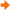Formulas and Functions (36 questions) Formating (29 questions) Charts (22 questions) Output: Setup and Print (24 questions) Functions (17 questions) Images (2 questions) LibreOffice Calc (BENCHMARK) (30 questions) LibreOffice Calc (ASSESSMENT) (30 questions)

 5384 Enter a function in cell C9 of the SALES worksheet to return the sum of the cell range C5:C8.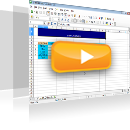5385 In cell L5 of the SALES worksheet enter a formula that returns the quotient of cell Ê5 divided by cell Ê9, so that if reproduced on the cell range L6:L9, the reference to cell K9 remains stable.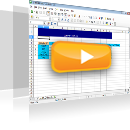5386 Enter a function in cell C10 that subtracts the value in cell C5 from the value in cell C9.5387 Enter the appropriate function in cell C14 to return the maximum sales for January (cell range C5:C8).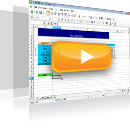5388 Enter a formula in cell C15 to return the word DELLA, if the content of cell C5 is equal to the content of cell C14; otherwise the formula should return the word other.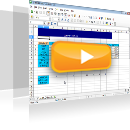5389 Correct the error of the formula in cell D20 of the 2ndhour2 worksheet.5390 At the SALES worksheet, change the formula to the cell D27 so that if copied or filled in automatically to all cells of the cell range D27:Ê31, it should calculate correctly the hypothetical turnover for each way of payment. Lastly, apply this formula to this cell range.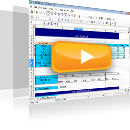5391 Given that sales equal to beginning pieces + supplies - end pieces - destroyed pieces, enter a formula in the cell range F7:F9 that returns the sales for each product. Enter a formula in the cell range H7:H9 that returns the sales value in Drachmas and use the result to calculate the sales value in Euros in the cell range I7:I9. (the euro-drachma exchange rate is provided in cell G2)5392 Reproduce the function of cell C9 to the cell range D9:K9 of the SALES worksheet.5393 Change the function in cell B4, so that the reference to cell A1 uses an absolute reference only by column.5394 Change the function in cell B4, so that the reference to cell A1 is absolute only by row.5395 Enter a function in cell A1 that counts non blank cells for the cell range A3:A15000. Also, enter a function in cell C116 that returns the number of cells displaying a numerical content for the cell range C3:C110 and another function to return non blank cells for the same cell range in cell C117.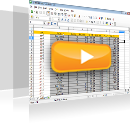5396 Enter a function that returns in cell E116 the average cost of the products displayed in the cell range Å3:Å110.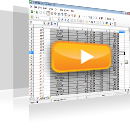5397 Enter a function that returns in cell E116 the minimum cost for the cell range E3:E110 and another function that returns in cell E117 the maximum cost for the same cell range.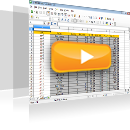5398 Enter the appropriate function in cell B1, so that if the value in cell (A1) is higher than 5000, the function will return 1. If the value in cell A1 is less than or equal to 5000, the function will return 0. Reproduce the function up to cell B10.5399 Insert the appropriate function in cell E1, so that if the value in cell A1 is higher than the value in cell B1, the function will return the value of cell C1, and if not, the function will return the value of cell D1. Reproduce this function up to cell E10.5400 There is a message for an error in cell G6. The formula doesn’t contain any errors. Without changing cell G6, find out which of the cell causes the error and insert the appropriate formula in it.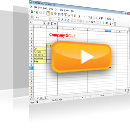5401 Make sure the indication #### in cell D7 is not displayed.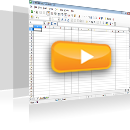5402 Automatically fill in the cell range A2:A1000 using the text appearing in cell A1.5403 Cell G9 displays the cost of the RAM memory in euros. Calculate the cost of the RAM memory in dollars in cell H9 taking into account the euro to dollar exchange rate displayed in cell E19. Then reproduce the formula in the cell range H10:H17.5404 Enter a function in cell E116 to calculate the minimum value appearing in the cell range Å3:Å110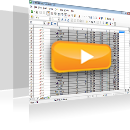5405 Insert a function in cell C116 to count the total of cells containing numbers within the cell range C3:C110.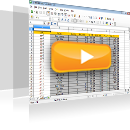5406 Insert a function in cell A1 to count non blank cells within the cell range A3:A15000.5485 Insert the appropriate function in the cell range B1:B50 that rounds the values of column A to 4 decimal places. Insert the appropriate function in the cell range C1:C50 that rounds the values of column A to 2 decimal places. Insert the appropriate function in the cell range D1:D50 that rounds the values of column A to the left of the decimal point.5494 Use the appropriate function in cell J4 to display the lowest value in the cell range D5:D10.5495 Navigate to cell D12 and calculate the maximum value of the cell range D5:G10.5496 Navigate to cell J4 and use the appropriate function to display the maximum value of the cell range F5:F10.5497 Navigate to cell G13 and use the appropriate function to display the sum of the values appearing in the cell range G5:G10. Then, save changes.5498 Navigate to cell H17 of the active worksheet and use the appropriate function to display the total of the cell range H6:H9.5499 Navigate to cell E11 of the SALES worksheet and use the appropriate function to display the sum of the values displayed in the cell range E6:E9.5500 Use the appropriate function in cell E11, to return the day of the date displayed in cell E4.5501 Use the appropriate function in cell E14 to round the contents of cell E11 to two decimal places.5502 Use the countblank function in cell B1 to count the blank cells in the cell range A1:A400.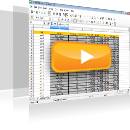12016 In order to apply an absolute reference only by column in cell B15, which of the following forms formulas is the correct one?12017 The correct way to enter a formula is displayed in cell…12018 Which of the following formulas is the correct one in order to apply the correct absolute reference in cell D6?